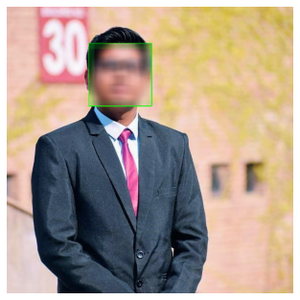Related Articles

# How to Blur Faces in Images using OpenCV in Python?

• Last Updated : 24 Jan, 2021

Prerequisite: OpenCV

OpenCV is a huge open-source library for computer vision, machine learning, and image processing.  It can process images and videos to identify objects, faces, or even the handwriting of a human. When it is integrated with various libraries, such as Numpy which is a highly optimized library for numerical operations, then the number of weapons increases in your Arsenal i.e whatever operations one can do in Numpy can be combined with OpenCV.

This article discusses how a face in an image can be blurred using OpenCV.

Requirements:

Apart from OpenCV module, to obtain this functionality we also need Haar Cascade frontal-face classifier needs to be downloaded. It is available as XML file and is used for detecting faces in an image

Approach

• Import module
• Reading an image using OpenCV
• Plotting it
• Detect face
• Draw a rectangle on the detected face
• Blur the rectangle
• Display output

Below is the implementation.

Input image:Original: my_img.jpg

## Python3

 `# Importing libraries``import` `numpy as np``import` `cv2``import` `matplotlib.pyplot as plt`` ` `# A function for plotting the images`` ` ` ` `def` `plotImages(img):``    ``plt.imshow(img, cmap``=``"gray"``)``    ``plt.axis(``'off'``)``    ``plt.style.use(``'seaborn'``)``    ``plt.show()`` ` ` ` `# Reading an image using OpenCV``# OpenCV reads images by default in BGR format``image ``=` `cv2.imread(``'my_img.jpg'``)`` ` `# Converting BGR image into a RGB image``image ``=` `cv2.cvtColor(image, cv2.COLOR_BGR2RGB)`` ` `# plotting the original image``plotImages(image)`` ` `face_detect ``=` `cv2.CascadeClassifier(``'haarcascade_frontalface_alt.xml'``)``face_data ``=` `face_detect.detectMultiScale(image, ``1.3``, ``5``)`` ` `# Draw rectangle around the faces which is our region of interest (ROI)``for` `(x, y, w, h) ``in` `face_data:``    ``cv2.rectangle(image, (x, y), (x ``+` `w, y ``+` `h), (``0``, ``255``, ``0``), ``2``)``    ``roi ``=` `image[y:y``+``h, x:x``+``w]``    ``# applying a gaussian blur over this new rectangle area``    ``roi ``=` `cv2.GaussianBlur(roi, (``23``, ``23``), ``30``)``    ``# impose this blurred image on original image to get final image``    ``image[y:y``+``roi.shape[``0``], x:x``+``roi.shape[``1``]] ``=` `roi`` ` ` ` `# Display the output``plotImages(image)`

Output:Blurred image

Attention geek! Strengthen your foundations with the Python Programming Foundation Course and learn the basics.

To begin with, your interview preparations Enhance your Data Structures concepts with the Python DS Course. And to begin with your Machine Learning Journey, join the Machine Learning – Basic Level Course

My Personal Notes arrow_drop_up